# Get Last Name From Full Name

This post will guide you how to extract the last name within a Full name in excel. You need to use a combination of the RIGHT function, the LEN function, the FIND function and the SUBSTITUTE function to create a complex formula in excel.

## Get Last Name From Full Name

You can use the below generic formula to achieve it:

`=RIGHT( B1, LEN(B1) - FIND( "#", SUBSTITUTE( B1," ","#", LEN(B1) - LEN( SUBSTITUTE( B1, " ","")))) )`

​Let’s see how the above formula works:

If you want to get the last name from a full name separated by the space character, you need to get the length of the last name or get the position of the last space character in Cell B1. Then using the RIGHT function to extract the last name based on the starting position returned by the FIND function.

how to get the position of the last space character, you need to use the formula as follows:

`=FIND( "#", SUBSTITUTE( B1," ","#", LEN(B1) - LEN( SUBSTITUTE( B1, " ",""))))`

The Len(B1) returns the length of the full name in Cell B1

`=LEN(B1)`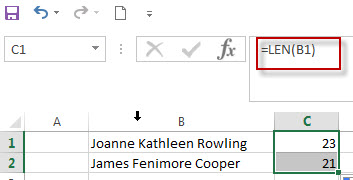The second SUBSTITUTE function will remove all of space character, and its returned result is used to by the LEN function to get the length of full name without space character.

`=LEN( SUBSTITUTE( B1, " ",""))`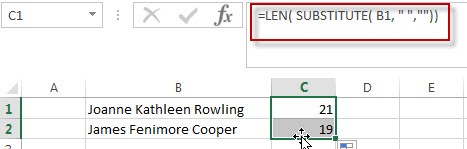The length of the full name without any spaces characters is subtracted from the length of the full name, then you will get that how many spaces in the full name.

`=LEN(B1) - LEN( SUBSTITUTE( B1, " ",""))`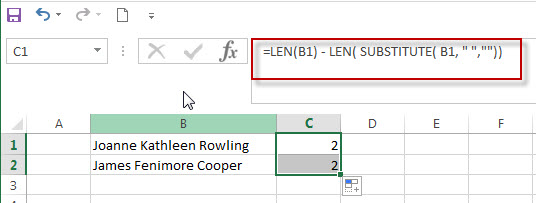If we get the result by the above formula as 2, it means that there are 2 spaces character in the full name.  Then we need to convert the second space character to hash sign using the first SUBSTITUTE function.

`=SUBSTITUTE( B1," ","#", LEN(B1) - LEN( SUBSTITUTE( B1, " ","")))`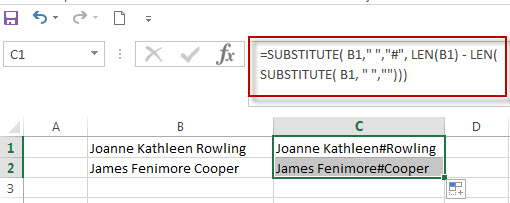The FIND function will locate the hash sign within the string returned by the above SUBSTITUTE function. And it is also the position of the last space character in the full name.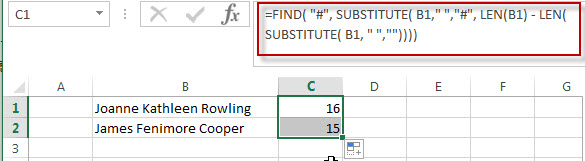Next, we need to subtract the numeric position of the last space character by the length of full name to get the length of the last name. It will be used by the RIGHT function to extract the last name:

`=RIGHT( B1, LEN(B1) - FIND( "#", SUBSTITUTE( B1," ","#", LEN(B1) - LEN( SUBSTITUTE( B1, " ","")))) )`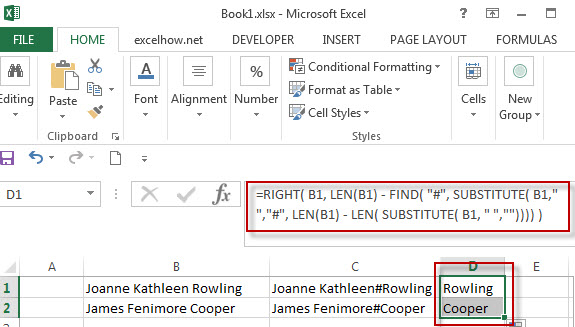### Related Formulas

• Get First Name From Full Name
If you want to get the first name from a full name list in Column B, you can use the FIND function within LEFT function in excel. The generic formula is as follows:=LEFT(B1,FIND(” “,B1)-1).…

### Related Functions

• Excel Find function
The Excel FIND function returns the position of the first text string (substring) from the first character of the second text string.The FIND function is a build-in function in Microsoft Excel and it is categorized as a Text Function.The syntax of the FIND function is as below:= FIND  (find_text, within_text,[start_num])…
• Excel SUBSTITUTE function
The Excel SUBSTITUTE function replaces a new text string for an old text string in a text string.The syntax of the SUBSTITUTE function is as below:= SUBSTITUTE  (text, old_text, new_text,[instance_num])…
• Excel LEN function
The Excel LEN function returns the length of a text string (the number of characters in a text string).The LEN function is a build-in function in Microsoft Excel and it is categorized as a Text Function.The syntax of the LEN function is as below:= LEN(text)…
• Excel RIGHT function
The Excel RIGHT function returns a substring (a specified number of the characters) from a text string, starting from the rightmost character.The syntax of the RIGHT function is as below:= RIGHT (text,[num_chars])…